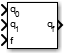# Quaternion Interpolation

Quaternion interpolation between two quaternions

• Library:
• Aerospace Blockset / Utilities / Math Operations

•## Description

The Quaternion Interpolation block calculates the quaternion interpolation between two normalized quaternions by an interval fraction. Aerospace Blockset™ uses quaternions that are defined using the scalar-first convention. Select the interpolation method from SLERP, LERP, or NLERP. For equations used for the interpolation methods, see Algorithms.

The two normalized quaternions are the two extremes between which the block calculates the quaternion.

## Ports

### Input

expand all

First normalized quaternion for which to calculate the interpolation. This quaternion must be a normalized quaternion

Data Types: `double`

Second normalized quaternion for which to calculate the interpolation, specified as a 4-by-1 vector or 1-by-4 vector. This quaternion must be a normalized quaternion.

Data Types: `double`

Interval fraction by which to calculate the quaternion interpolation . This value varies between 0 and 1. It represents the intermediate rotation of the quaternion to be calculated. This fraction affects the interpolation method rotational velocities.

#### Dependencies

The interval fraction affects the rotational velocities of the interpolation methods for the Methods parameter. For more information on interval fractions, see .

Data Types: `double`

### Output

expand all

Natural logarithm of quaternion, returned as a vector.

Data Types: `double`

## Parameters

expand all

Quaternion interpolation method to calculate the quaternion interpolation, specified as:

• `SLERP`

Quaternion slerp. Spherical linear quaternion interpolation method.

• `LERP`

Quaternion lerp. Linear quaternion interpolation method.

• `NLERP`

Normalized quaternion linear interpolation method.

#### Dependencies

These methods have different rotational velocities, depending on the interval fraction from input port f. For more information on interval fractions, see .

#### Programmatic Use

 Block Parameter: `method` Type: character vector Values: `'SLERP'` | `'LERP'` | `'NLERP'` Default: `'SLERP'`

Out-of-range block behavior, specified as follows.

ActionDescription

`None`

No action.

`Warning`

Warning in the MATLAB® Command Window, model simulation continues.

`Error` (default)

MATLAB returns an exception, model simulation stops.

#### Programmatic Use

 Block Parameter: `action` Type: character vector Values: `'None'` | `'Warning'` | `'Error'` Default: `'Error'`

## Algorithms

$Slerp\left(p,q,h\right)=p{\left({p}^{*}q\right)}^{h}$ with $h\in \left[0,1\right].$

$LERP\left(p,q,h\right)=p\left(1-h\right)+qh$ with $h\in \left[0,1\right].$

With $r=LERP\left(p,q,h\right),$$NLERP\left(p,q,h\right)=\frac{r}{|r|}.$

 Dam, Erik B., Martin Koch, Martin Lillholm. "Quaternions, Interpolation, and Animation." University of Copenhagen, København, Denmark, 1998.

## Support